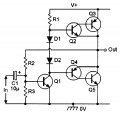# complementary pairs of unity-gain common-emitter amplifiers with 100% negative feedback

#### halil06behzat

Joined Apr 17, 2017
8
Guys I had a problem before on this topic Thanks to everyone But I have more question this time.I have to analyze theoreticallyThats my school project
First I cant obtain emitter current value or base current value theoretically by using KCL or KVL .How to I obtain these values theoretically.Second How can I make SSAC(Small signal analyze) and AC Analyze.Because there is no resistor around sziklai pairs so I cant obtain loop then I cant determine Rac(AC resistor value).Last edited by a moderator:

#### Jony130

Joined Feb 17, 2009
5,405
What did you do so far to obtain the DC operation point? The supply voltage is? What are the resistor values? And the BJT types or parameters you have been given?

#### Jony130

Joined Feb 17, 2009
5,405
OK, what transistor "model" you have been using when you solve the DC operation point? Simply "beta" model (Ic = β*Ib and fixed Vbe) or Shockley equation?

#### halil06behzat

Joined Apr 17, 2017
8
ho shockley equation
I assumad Vbe=0.7 theoretically and beta for TIP31=38.07 and TIP32=18.74 I obtained these values on proteus so I assumed like tibs way

#### MrAl

Joined Jun 17, 2014
9,344
Hello,

I think you can handle the two output transistors as voltage followers, so if you are allowed to use the approximation of 0.7 volts for diodes, i think you can figure this out pretty quick because it's mostly about adding up voltage drops, as long as the beta works with the current demand, which we can probably assume it does.
We could probably also assume the output is biased to 1/2 of Vcc cause that's the usual way of doing it which then gives equal positive and negative output voltage swings.
So try working from the output towards the input, see what you get.
Hint: Q2 base follows it's emitter, the diodes follow Q2 base, the collector of Q1 follows the two diodes, the Q4 base follows Q1 collector, Q4 emitter follows the output. So it's one big voltage drop loop where we can probably assume all the drops are constant as a first approximation. Q1 collector controls all that.

BTW this does not have 100 percent negative feedback. The feedback comes roughly from the ratio of R2 and R3 so we get only partial feedback.

Last edited:

#### halil06behzat

Joined Apr 17, 2017
8
thanks I considered your words.Meanwhile,who can draw small signal equivalent of this circuit.I cant draw it Because five transistors confused my mind.So far I examine 1 or two transistor circuits.I cant analyze with 5 transistors.

#### halil06behzat

Joined Apr 17, 2017
8
one more thing I cant calculate current gain and voltage gain.How can Idetermine this values

#### MrAl

Joined Jun 17, 2014
9,344
Hello,

An approximate analysis for the gain could go like this...

For the gain you could probably just assume the output is biased to 1/2 of Vcc.
That means the top of D1 is at Vcc/2+0.7, and that means that the current through R1 is:
iR1=(Vcc/2-0.7)/R1

and with a given assumed Beta for Q1, the base current would be:
iBQ1=iR1/BetaQ1

With Vcc/2 at the top of R2 and the Vbe of Q1 about 0.7v, the current through R2 is:
iR2=(Vcc/2-0.7)/R2

The current through R3 is then:
iR3=iR2-iBQ1

and the equivalent resistance of the base emitter of Q1 is:
rBEQ1=0.7/iBQ1

Considering the input cap to be a short circuit when a small voltage dv is applied to the input the base voltage rises by that amount dv so the increase in current into the base would be:
di=dv/rBEQ1

and this in turn changes the collector voltage because of the increase in current through R1, and that directly changes the output voltage. Since the output voltage will fall, the voltage at the top of R2 will fall and that is the negative feedback. You then have to come up with an equation to express this action, which will be similar to any other feedback amplifier equation.

See what you can do from here.

Last edited:

#### halil06behzat

Joined Apr 17, 2017
8
thanks
In ac equivalent circuit the r0 value(r not) can be ignored?
Can I dont take the r0 value.Please answer quickly I dont have enough time

#### MrAl

Joined Jun 17, 2014
9,344
Hi again,

I am not sure what resistor you are talking about here.
Sometimes these discussions take time because we have to sleep and other things•absf

#### hobbyist

Joined Aug 10, 2008
890
For the gain you could probably just assume the output is biased to 1/2 of Vcc.
That means the top of D1 is at Vcc/2+0.7, and that means that the current through R1 is:
iR1=(Vcc/2+0.7)/R1

.
Wouldn't it be [Vcc - ((Vcc/2) +0.7) / R1] = iR1. ?

#### MrAl

Joined Jun 17, 2014
9,344
Wouldn't it be [Vcc - ((Vcc/2) +0.7) / R1] = iR1. ?

Hi,

Yes typo on my part. It should have been
iR1=(Vcc/2-0.7)/R1

which is the same thing. It's fixed now, thanks for mentioning this.